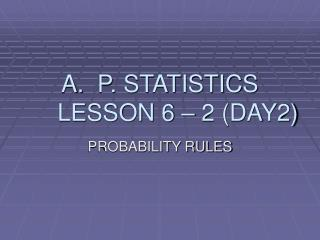DownloadDownload PresentationP. STATISTICS LESSON 6 – 2 (DAY2)

P. STATISTICS LESSON 6 – 2 (DAY2)

Télécharger la présentationP. STATISTICS LESSON 6 – 2 (DAY2)

- - - - - - - - - - - - - - - - - - - - - - - - - - - E N D - - - - - - - - - - - - - - - - - - - - - - - - - - -
Presentation Transcript

1. P. STATISTICSLESSON 6 – 2 (DAY2) PROBABILITY RULES

2. ESSENTIAL QUESTION: What are the probability rules and how are they used to solve problems? Objectives : • To become familiar with the probability rules. • To use the probability rules to solve problems in which they may be used.

3. Probability Rules • Rule 1 : The probability P(A) of any event A satisfies 0 ≤ P(A) ≤ 1. • Rule 2 : If S is the sample space in probability model, then P(S) = 1 • Rule 3 : The compliment of any event A is the event that A does not occur, written as Ac . The compliment rule states that P(Ac) = 1 – P(A) • Rule 4 : Two events A and B are disjoint ( also called mutually exclusive ) if they have no outcomes in common and so can never occur simultaneously. If A and B are disjoint, P(A or B) = P(A) + P(B) This is the addition rule for disjoint events.

4. Set Notation A U B – read “ A union B” is the set of all outcomes that are either in A or B’ Empty event – The event that has no outcomes in it. (ø) If two events A and B are disjoint (mutually exclusively), we can write A ∩ B = ø, read “intersect B is empty.” A picture like that shows the Sample space S as a rectangular area and events as areas within S is called a Venn diagram.

5. Venn Diagram The events A and B are disjoint because they do not overlap; S B A

6. Compliment Ac The compliment Ac contains exactly the outcomes not in A. Note that we could write A U Ac = A ∩ B = ø. A Ac

7. Example 6.8 • Page 344

8. Example 6.9 • Page 344 probabilities for rolling dice.

9. Assigning probabilities: finite number of outcomes • PROBABILITIES IN A FINITE SAMPLE SPACE Assign a probability to each individual outcome. These probabilities must be between numbers 0 and 1 must have sum 1. The probability of any event is the sum of probabilities of the outcomes making up the event.

10. Benford’s Law • Page 345 example 6.10 Used in accounting as a test for faked numbers in tax returns, payment records, invoices, expense account claims, and many other settings often display patterns that aren’t present in legitimate records. Usually applies to first digits.

11. Assigning probabilities: equally likely outcomes • Assigning correct probabilities to individual outcomes often requires long observation of the random phenomenon. In some special circumstances, however, we are willing to assume that individual outcomes are equally likely because of some balance in the phenomenon.

12. Equally likely Outcomes If a random phenomenon has k possible outcomes, all equally likely, then each individual outcome has probability 1/k. The probability of any event A is P(A) = count of outcomes in A = count of outcomes in A Count of outcomes in S k

13. The Multiplication Rule for Independent Events Rule 5 : Two events A and B are independent if knowing that one occurs does not change the probability that the other occurs. If A and B are independent, P(A and B) = P(A)P(B) This is the multiplication rule for independent events.

14. Venn diagram of independent events A and B A B

15. Independent and Disjoint Disjoint – Mutually exclusive Independent – The outcome of one trial must not influence the outcome of any other. Unlike disjointness or compliments, independence cannot be pictured by a Venn diagram, because it involves the probabilities of the events rather than just the outcomes that make up the events.